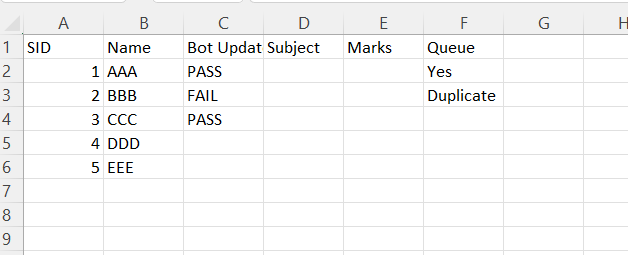# Data table merge in uipath

Hi Team, i have two tables merge to specific column and cell

My expect output is below

With out using for each activity pls give any solution.

@AJITH_SK

What if bot_update have values in both the tables ,which is to be picked?

ideally we can use a join Datatable activity

cheers

Read the first and Second DataTable called `dtTable1` and `dtTable2`
Create a variable called mergedData (Variable type shlould be IEnumerable)

Use assign activity

mergedData = From table1 In dtTable1.AsEnumerable()
Join table2 In dtTable2.AsEnumerable()
On table1.Field(Of String)(“SID”) Equals table2.Field(Of String)(“SID”)
table1.Field(Of String)(“SID”),
table1.Field(Of String)(“Name”),
table2.Field(Of String)(“Queue”)
}, False)

Create one variable called dtMerged

Use assign activty

dtMerged As DataTable = mergedData.CopyToDataTable()

Hope this hells

Hi @AJITH_SK ,
If 2 data table are not big, you can use for each row in data table, write dt2 to dt1 by “SID”
and row is blank
or they are big, you can try LNQ
regards,

@AJITH_SK

you can try by using invoke code activity

``````Dim counter As int32
For Each row As datarow In dt1.AsEnumerable
row("Queue")=dt2.Rows(counter).Item("Queue")
If row(2).ToString="" Then
row(2)=dt2.Rows(counter).item(1)
Exit For
End If
counter=counter+1
Next
``````

@AJITH_SK

For Each row1 As datarow In dt1.AsEnumerable()
For Each row2 As datarow In dt2.AsEnumerable()
If row1(“SID”).ToString.Equals(row2(“SID”).ToString) Then
row2(“Queue”)=row1(“Queue”)
Exit For
End If
Next row2
Next row1

through invoke Code

Hi @Shiva_Nikhil , i tried above code , its not working after 2 rows,
below mentioned screen shottable 2 data merge to table 1.only bot updates column and Queue

@AJITH_SK

``````Dim counter As int32
For Each row As datarow In dt1.AsEnumerable
row("Queue")=dt2.Rows(counter).Item("Queue")
If row(2).ToString="" Then
row(2)=dt2.Rows(counter).item(1)
counter=counter+1
Else
row(2)=row(2).ToString
counter=counter+1
End If
Next

``````

Try this once

1 Like

@AJITH_SK

Try these in invoke code activity and send dt1 and dt2 as in/out and in arguments respectively

`dt1.AsEnumerable.ToList.ForEach(sub(r) r("Bot Updates") = If(r("Bot Updates").ToString,r("Bot Updates").ToString,dt2.AsEnumerable.Where(function(x) x("SID").ToString.Equals(r("SID").ToString))(0)("Bot Updates").ToString))`

`dt1.AsEnumerable.ToList.ForEach(sub(r) r("Queue") = dt2.AsEnumerable.Where(function(x) x("SID").ToString.Equals(r("SID").ToString))(0)("Queue").ToString)`

cheers

1 Like

It is IEnumerable DataRow

1 Like

This topic was automatically closed 3 days after the last reply. New replies are no longer allowed.